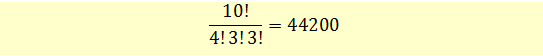# Multiplication Rule of Counting

Counting problems have to do with counting the total number of outcomes or logical possibilities of something. For example, if we have to flip a coin, we can easily count the number of outcomes. There are only two possible outcomes, either heads or tails. However, as our problem or data set becomes large and complex, so does the total number of possible outcomes. Counting the outcomes one by one may not be possible then and we will have to use some techniques to make our job easy. In this section we will look at a few such techniques.

### Multiplication Rule of Counting

Problem 1

If there are A ways of doing something and B ways of doing another thing, then the total number of ways to do both the things is = A x B.

For example, assume that your investment process involves two steps. The first step can be done in two ways and the second step can be done in three ways. There are total 2x3 = 6 ways of carrying out both the steps.

Problem 2: Arranging Items in a group.

Suppose we have a group of 5 people and we want them to stand in a queue. In the queue the first position can be filled in 5 ways. Now position 1 is filled and we have 4 more people left. The second position can be filled in 4 ways. Similarly, third position can be filled in 3 ways and so on. The total no. of ways these 5 positions can be filled is:

\= 5 * 4 * 3 * 2 * 1 = 120

If the number of people was n, then this can be written as

n! = n (n-1)(n-2)(n-3)…1

n! is known as factorial.

Solving n factorial using BA II Plus calculator

Suppose you want to calculate 5!. To solve this on your calculator, press 5[2ND]x!.

### Multinomial Formula (General Formula for Labelling)

The factorial formula above assumed only one group. However, we may have labelling problems with multiple groups. For example, suppose that we have a group of 10 stocks and we want to label four of these stocks as BUY, three stocks as SELL and 3 stocks as HOLD. What is the total number of ways to do this?

This problem can be solved using the general formula for labelling.We have k different labels, n1, n2,…nk of each type.

In our example,

n2 = SELL = 3

n3 = HOLD = 3

So, the 10 stocks can be labelled in number of ways:Get our Data Science for Finance Bundle for just $29$51.